#### Nonlocal formulation

Nonlocal formulation of the MDM model is based on the averaging of the inverse integrity tensor. This roughly corresponds to the nonlocal isotropic damage model with averaging of the compliance variable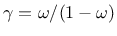, which does not cause any spurious locking effects. In equation (81) for the evaluation of the damage effect tensor, the inverse integrity tensor is replaced by its weighted average with components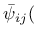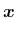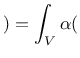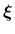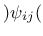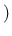d(87)

By fitting a wide range of numerical results, it has been found that the parameters of the nonlocal MDM model can be estimated from the measurable material properties using the formulas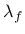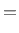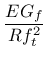(88)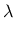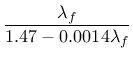(89)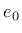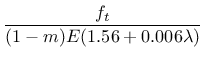(90)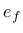(91)

where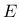is Young's modulus,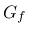is the fracture energy,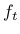is the uniaxial tensile strength,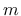is the compressive correction factor, typically chosen as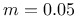, andis the radius of nonlocal interaction reflecting the internal length of the material.
Borek Patzak
2019-03-19